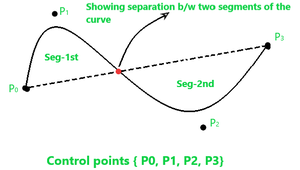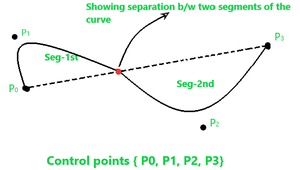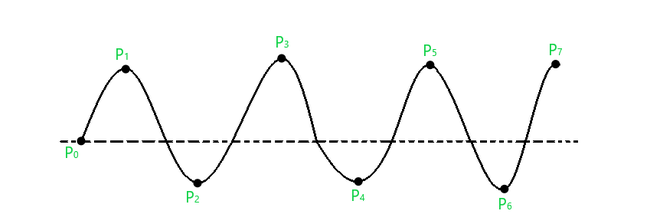B-Spline Curve in Computer Graphics

• Difficulty Level : Easy
• Last Updated : 22 Jan, 2021

Prerequisite – Bezier Curve

Concept of B-spline curve came to resolve the disadvantages having by Bezier curve, as we all know that both curves are parametric in nature. In Bezier curve we face a problem, when we change any of the control point respective location the whole curve shape gets change. But here in B-spline curve, the only a specific segment of the curve-shape gets changes or affected by the changing of the corresponding location of the control points.

In the B-spline curve, the control points impart local control over the curve-shape rather than the global control like Bezier-curve.

B-spline curve shape before changing the position of control point P1B-spline curve shape after changing the position of control point P1You can see in the above figure that only the segment-1st shape as we have only changed the control point P1, and the shape of segment-2nd remains intact.

B-spline Curve :
As we see above that the B-splines curves are independent of the number of control points and made up of joining the several segments smoothly, where each segment shape is decided by some specific control points that come in that region of segment. Consider a curve given below –Attributes of this curve are –

• We have “n+1” control points in the above, so, n+1=8, so n=7.
• Let’s assume that the order of this curve is ‘k’, so the curve that we get will be of a polynomial degree of “k-1”. Conventionally it’s said that the value of ‘k’ must be in the range: 2 ≤ k ≤ n+1. So, let us assume k=4, so the curve degree will be k-1 = 3.
• The total number of segments for this curve will be calculated through the following formula –
Total no. of seg = n – k + 2 = 7 – 4 + 2 = 5.

Knots in B-spline Curve :
The point between two segments of a curve that joins each other such points are known as knots in B-spline curve. In the case of the cubic polynomial degree curve, the knots are “n+4”. But in other common cases, we have “n+k+1” knots. So, for the above curve, the total knots vectors will be –

Total knots = n+k+1 = 7 + 4 + 1 = 12

These knot vectors could be of three types –

• Uniform (periodic)
• Open-Uniform
• Non-Uniform

B-spline Curve Equation : The equation of the spline-curve is as follows –Where Pi, k, t correspondingly represents the control points, degree, parameter of the curve.And following are some conditions for xi are as follows –Some cases of Basis function :Properties of B-spline Curve :

• Each basis function has 0 or +ve value for all parameters.
• Each basis function has one maximum value except for k=1.
• The degree of B-spline curve polynomial does not depend on the number of control points which makes it more reliable to use than Bezier curve.
• B-spline curve provides the local control through control points over each segment of the curve.
• The sum of basis functions for a given parameter is one.
My Personal Notes arrow_drop_up